# Recurrence And Searching MCQ - 2

## 15 Questions MCQ Test RRB JE for Computer Science Engineering | Recurrence And Searching MCQ - 2

Description
This mock test of Recurrence And Searching MCQ - 2 for Computer Science Engineering (CSE) helps you for every Computer Science Engineering (CSE) entrance exam. This contains 15 Multiple Choice Questions for Computer Science Engineering (CSE) Recurrence And Searching MCQ - 2 (mcq) to study with solutions a complete question bank. The solved questions answers in this Recurrence And Searching MCQ - 2 quiz give you a good mix of easy questions and tough questions. Computer Science Engineering (CSE) students definitely take this Recurrence And Searching MCQ - 2 exercise for a better result in the exam. You can find other Recurrence And Searching MCQ - 2 extra questions, long questions & short questions for Computer Science Engineering (CSE) on EduRev as well by searching above.
QUESTION: 1

### Consider the following recurrence: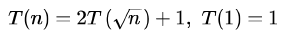Which one of the following is true?

Solution: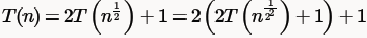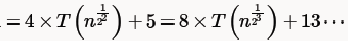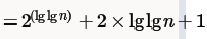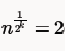( Putting 2so that we can take log. one more step of recurrence can't change the complexcity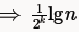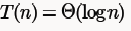QUESTION: 2

### Let Xn denote the number of binary strings of length n that contain no consecutive 0s. Which of the following recurrences does Xn satisfy?

Solution:

0 1 -2
01 10 11 -3
010 011 101 110 111 -5
0101 0110 0111 1010 1011 1101 1110 1111 -8

So, xn = xn-1 + xn-2 (For all the strings ending in 1, we get two new strings and for all strings ending in 0, we get a new string. So, the new set of strings for n+1, will have exactly n strings ending in 1)

x5 = 8+5 = 13

QUESTION: 3

### Let Xn denote the number of binary strings of length n that contain no consecutive 0s. x5 = 8+5 = 13

Solution:

Number of binary strings of length n that contain no consecutive 0s, following will be the required recurrence relation:

T(n) = T(n-1) + T(n-2) n>2

base condition T(1) = 2 and T(2) = 3

T(1) =2 There will be 2 strings of length 1, i.e 0 & 1
T(2) =3 There will be 3 strings of length 2, i.e. 01,10,11

T(3) = T(1) + T(2) = 2+3 = 5
T(4) = T(3) + T(2) = 5 + 3 = 8
T(5) = T(4) +T(3) = 8 + 5 = 13

QUESTION: 4

When n = 22k for some  k ≥ 0, the recurrence relation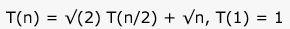evaluates to :

Solution: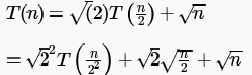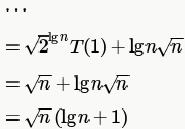If we use Master theorem we get option B. But one must know that Matser theorem is used to find the asymptotic bound and not an EXACT value. And in the question here it explicitly says "evaluates to".

QUESTION: 5

The running time of an algorithm is represented by the following recurrence relation: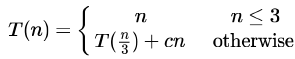Which one of the following represents the time complexity of the algorithm?

Solution: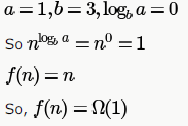To, check Master theorem case 3, we need C>0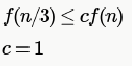So using case three of master theorem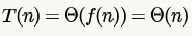QUESTION: 6

The recurrence relation capturing the optimal execution time of the Towers of Hanoi  problem with n discs is

Solution:

Recurrence relation for Towers of Hanoi is
T(1) = 1
T(n) = 2 T( n-1 ) +1

QUESTION: 7

Which one of the following correctly determines the solution of the recurrence relation with T(1) =1?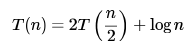Solution: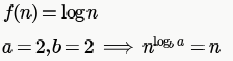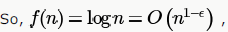we can take any ε from 0-1 for example 0.5 which gives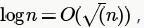whose proof
is given here: http://math.stackexchange.com/questions/145739/prove-that-logn-o-sqrtn

QUESTION: 8

Let an represent the number of bit strings of length n containing two consecutive 1s. What is the recurrence relation for an ?

Solution:

Counting the number of bit strings NOT containing two consecutive 1's. (It is easy to derive a recurrence relation for the NOT case as shown below.)

0 1
00 01 10 - 3 (append both 0 and 1 to any string ending in 0, and append 0 to any string ending in 1)

000 001 010 100 101 - 5 (all strings ending in 0 give two strings and those ending in 1 give 1 string)

0000 0001 0010 0100 0101 1000 1001 1010 - 8

....

an' = an-1' + an-2' (where an denote the number of bit strings of length n containing two consecutive 1s)

2n - an = (2n-1 - an-1) + (2n-2 - an-2)

an = 2n-2(4 - 2 - 1) + an-1 + an-2

an = an-1 + an-2 + 2n-2

QUESTION: 9

Consider the following recursive C function.

void get(int n)

{
if (n<1) return;

get (n-1);

get (n-3);

printf("%d", n);

}

If get(6) function is being called in main() then how many times will the get() function be invoked before returning to the main()?

Solution:

T(n) = T(n-1) + T(n-3) + 2, here T(n) denotes the number of times a recursive call is made for input n. 2 denotes the two direct recursive calls.

T(n<=0) = 0
T(1) = 2
T(2) = 4
T(3) = 6
T(4) = 10
T(5) = 16
T(6) = 24

So, answer is 24 + 1 call from main = 25.

QUESTION: 10

Consider the recurrence function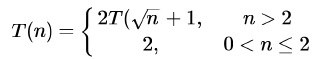Then T(n) in terms of θ notation is

Solution: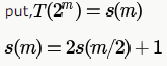Using case 1 of master method ,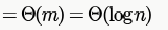QUESTION: 11

Consider the following recurrence relation: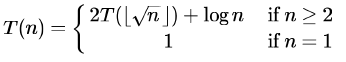Which of the following statements is TRUE?

Solution: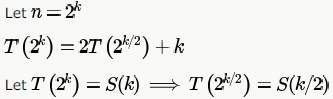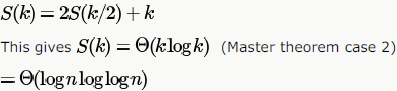QUESTION: 12

The average number of key comparisons required for a successful search for sequential search on n items is

Solution:

Expected number of comparisons

= 1x Probability of first element be x + 2 x Probability of second element be x + .... +n x Probability of last element be x.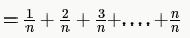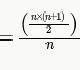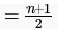QUESTION: 13

Consider the following C program that attempts to locate an element x in an array Y[ ] using binary search. The program is erroneous.

f (int Y , int x) {

int u, j, k;

i= 0; j = 9;

do {

k = (i+ j) / 2;

if( Y[k] < x) i = k; else j = k;

} while (Y[k] != x) && (i < j)) ;

if(Y[k] == x) printf(" x is in the array ") ;

else printf(" x is not in the array ") ;

}

On which of the following contents of Y and x does the program fail?

Solution:

when it is option C the control will continue to iterate as i=8 and j=9;

again and again i will be assigned k which itself equals 8 as 8+9/2 being stored in an integer type variable, will evaluate to 8

For option A, with X=9 , k will take the following values:

• 4
• 6
• 7
• 8 - y = 9, x found

For option D, with X = 10, k will take the following values:

• 4, y = 10, x found
QUESTION: 14

Consider the following C program that attempts to locate an element x in an array Y[ ] using binary search. The program is erroneous.

f (int Y , int x) {

int u, j, k;

i= 0; j = 9;

do {

k = (i+ j) / 2;

if( Y[k] < x) i = k;else j = k;

} while (Y[k] != x) && (i < j)) ;

if(Y[k] == x) printf(" x is in the array ") ;

else printf(" x is not in the array ") ;

}

The correction needed in the program to make it work properly is

Solution:

if( Y[k] < x) then i = k + 1;

if given element that we are searching is greater then searching will be continued upper half of array

otherwise j = k - 1;
lower half.
Take few case in consideration i.e.

1. all elements are same
2. increasing order with no repeatation
3. increasing order with repeatation.

QUESTION: 15

Suppose you are given an array A with 2n numbers.

The numbers in odd positions are sorted in ascending order, that is,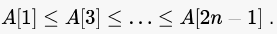The numbers in even positions are sorted in descending order, that is,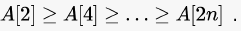What is the method you would recommend for determining if a given number is in the array?

Solution:

We can simply use clever indexing to binary search the element in the odd positions, and in the even positions separately.

This will take O(logn) time andO(1) space in the worst case.

A: Sorting using Quicksort will take O(n2) time.

B: Merging will take O(n) time and O(n) space.

C: Binary search only works on a sorted array.

E: Sequential search will take O(n) time.